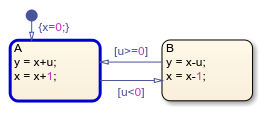Documentation

## Execute and Unit Test Stateflow Chart Objects

A standalone Stateflow® chart is a MATLAB® class that defines the behavior of a finite state machine. Standalone charts implement classic chart semantics with MATLAB as the action language. You can program the chart by using the full functionality of MATLAB, including those functions that are restricted for code generation in Simulink®. For more information, see Create Stateflow Charts for Execution as MATLAB Objects.

### Example of a Standalone Stateflow Chart

The file `sf_chart.sfx` contains the standalone Stateflow chart `sf_chart`. The chart has an local data `u`, `x`, and `y`.This example shows how to execute this chart from the Stateflow editor and in the MATLAB Command Window.

### Execute a Standalone Chart from the Stateflow Editor

To unit test and debug a standalone chart, you can execute the chart directly from the Stateflow editor. During execution, you enter data values and broadcast events from the user interface.

1. Open the chart in the Stateflow editor:

`edit sf_chart.sfx`

2. In the Symbols window, enter a value of `u` = 1 and click the icon. The editor creates a Stateflow chart object `my_sf_chart` and executes the default transition. The chart:

• Initializes `x` to the value of 0.

• Makes state `A` the active state.

• Assigns `y` to the value of 1.

• Increases the value of `x` to 1.

The chart animation highlights the active state `A`. The Symbols window displays the values `u` = 1, `x` = 1, and `y` = 1. The chart maintains its current state and local data until the next execution command.3. Click the iconagain. Because the value of `u` does not satisfy the condition `[u<0]` to transition out of state `A`, this state remains active and the values of `x` and `y` increase to 2. The Symbols window now displays the values `u` = 1, `x` = 2, and `y` = 2.4. In the Symbols window, enter a value of `u` = −1 and click the icon. The negative data value triggers the transition to state `B`. The Symbols window displays the values `u` = −1, `x` = 1, and `y` = 3.5. You can modify the value of any chart data in the Symbols window. For example, enter a value of `x` = 3. The chart will use this data value in the next time execution step.

6. Enter a value of `u` = 2 and click the icon. The chart transitions back to state `A`. The Symbols window displays the values `u` = 2, `x` = 4, and `y` = 5.7. To stop the chart animation and remove the Stateflow chart object `my_sf_chart` from the MATLAB workspace, click the icon.

To interrupt the execution and step through each action in the chart, add breakpoints before you execute the chart. For more information, see Debug a Standalone Stateflow Chart.

### Execute a Standalone Chart in MATLAB

You can execute a standalone chart in MATLAB without opening the Stateflow editor. If the chart is open, then the Stateflow editor highlights active states and transitions through chart animation.

1. Open the chart in the Stateflow editor. In the MATLAB Command Window, enter:

`edit sf_chart.sfx`

2. Create the Stateflow chart object by using the name of the `sfx` file for the standalone chart as a function. Specify the initial value for the data `u` as a name-value pair.

`s = sf_chart('u',1)`
```Stateflow Chart Execution Function y = step(s) Local Data u : 1 x : 1 y : 1 Active States: {'A'}```
This command creates the chart object `s`, executes the default transition, and initializes the values of `x` and `y`. The Stateflow editor animates the chart and highlights the active state `A`.3. To execute the chart, call the `step` function. For example, suppose that you call the `step` function with a value of `u` = 1:

`step(s,'u',1)`
`disp(s)`
```Stateflow Chart Execution Function y = step(s) Local Data u : 1 x : 2 y : 2 Active States: {'A'}```
Because the value of `u` does not satisfy the condition `[u<0]` to transition out of state `A`, this state remains active and the values of `x` and `y` increase to 2.4. Execute the chart again, this time with a value of `u` = −1:

`step(s,'u',-1)`
`disp(s)`
```Stateflow Chart Execution Function y = step(s) Local Data u : -1 x : 1 y : 3 Active States: {'B'}```
The negative data value triggers the transition to state `B`. The value of `x` decreases to 1 and the value of `y` increases to 3.5. To access the value of any chart data, use dot notation. For example, you can assign a value of 3 to the local data `x` by entering:

`s.x = 3`
```Stateflow Chart Execution Function y = step(s) Local Data u : -1 x : 3 y : 3 Active States: {'B'}```
The standalone chart will use this data value in the next time execution step.

6. Execute the chart with a value of `u` = 2:

`step(s,'u',2)`
`disp(s)`
```Stateflow Chart Execution Function y = step(s) Local Data u : 2 x : 4 y : 5 Active States: {'A'}```
The chart transitions back to state `A` and modifies the values of `x` and `y`.7. To stop the chart animation, delete the Stateflow chart object `s` from the MATLAB workspace:

`delete(s)`

### Execute Multiple Chart Objects

You can execute multiple chart objects defined by the same standalone chart. Concurrent chart objects maintain their internal state independently, but remain associated to the same chart in the editor. The chart animation reflects the state of the chart object most recently executed. Executing multiple chart objects while the Stateflow editor is open can produce confusing results and is not recommended.

## Related Topics

##### SupportGet trial now